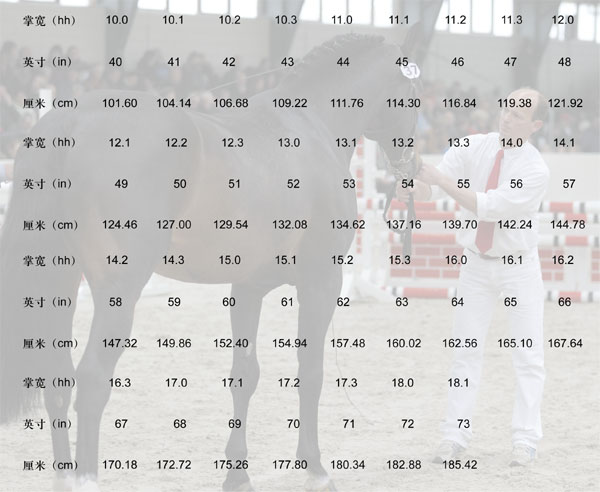## How to measure a horse by hands

2023-06-12 10:16

How to Measure a horse By Hands

When we choose a Horse Rug & Horse blanket, obviously we must check our horse size firstly ,usually we measure by CM, Feet, by Inch, quite often we measure by hands too .Hands here is the representation of palm width

The letter H represents the palm "Hands", or HH is "palm high" (hands high).

Criteria for distinguishing between pony and pony

The distinction between the pony (Pony) and the horse (Horse) worlds is divided by the height of the palm width, not by centimeters. The standard for a pony is less than 14.2hh, and someone higher than that is called a horse. Some countries may differ slightly, such as the Australian standard is 14.0hh.

Hand and foot

The British length unit "palm width" is used to measure the height of a horse, and another unit "foot", the length of a foot (foot), is the standard used to measure shoes, and obviously these are obviously a natural scale.

1 palm width (hands) =4 inches (inches)=10.16cm

1 foot (foot) =12 inches (inches)=30.48cm

Inch is also a "natural" unit, originally an Anglo-Saxon length, from the length of three large grains (horses like to eat barley), about 2.54cm.

A correct representation of the palm width

Here are a few examples, please keep in mind the "palm width" before the decimal point and the "inches" after the decimal point.

15.0hh (Or writing 15 hh) —— represents the height of a horse with 15 palm width.

15.1hh—— Represents a horse with 15 palm width, plus a height of 1 inch.

15.2hh—— Represents a horse with 15 palm width, plus a height of 2 inches.

15.3hh—— Represents a horse with 15 palm width, plus a height of 3 inches.

15.4hh—— This is wrong to write, because 1 palm width is equal to 4 inches, should carry, write 16.0hh.

15.5hh—— This is wrong, if you mean 15 plus 0.5, the correct way is 15.2hh.

How to convert?

14.2hh How to convert it into centimeters, there are two ways:

(1) Use palm width before the decimal point and inch after the decimal point.1 palm width = 10.16cm,14×10.16=142.24, plus 2 inches, 1 inch is equal to 2.54cm, then 2 inches is equal to 5.08cm. So the 14.2hh=147.32cm.

(2) All converted into inches, 1 palm width is equal to 4 inches, 14 palm width is equal to 56 inches, plus 2 inches, a total of 58 inches, 1 inch is equal to 2.54cm, so 14.2hh=147.32cm.

If the faction occurs, the basic principle is the same:

14.1-3/4hh This value is between 14.1 and 14.2, first converted to inch: 14×4+1.75=57.75 (inch), converted to 146.69cm.

20.2-3/4hh, or 20 palms, 2.75 inches, 20×4+2.75=80.75 inches, or 210.19cm.

Palm width, inch, cm conversion meterGet the latest price? We'll respond as soon as possible(within 12 hours)